# Folklore

## P vs. BPP ★★★

Author(s): Folklore

Conjecture   Can all problems that can be computed by a probabilistic Turing machine (with error probability < 1/3) in polynomial time be solved by a deterministic Turing machine in polynomial time? That is, does P = BPP?

Keywords: BPP; circuit complexity; pseudorandom generators

## Shuffle-Exchange Conjecture ★★★

Author(s): Beneš; Folklore; Stone

Given integers, letbe the smallest integersuch that the symmetric groupon the set of all words of lengthover a-letter alphabet can be generated as(times), where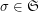is the shuffle permutation defined by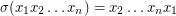, andis the exchange group consisting of all permutations inpreserving the first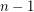letters in the words.

Problem  (SE)   Evaluate.
Conjecture  (SE), for all.

Keywords:

## Shuffle-Exchange Conjecture (graph-theoretic form) ★★★

Author(s): Beneš; Folklore; Stone

Given integers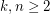, the 2-stage Shuffle-Exchange graph/network, denoted, is the simple-regular bipartite graph with the ordered pair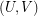of linearly labeled parts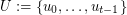and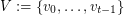, where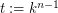, such that verticesand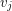are adjacent if and only if(see Fig.1).

Given integers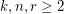, the-stage Shuffle-Exchange graph/network, denoted, is the proper (i.e., respecting all the orders) concatenation ofidentical copies of(see Fig.1).

Letbe the smallest integer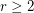such that the graphis rearrangeable.

Problem   Find.
Conjecture.

Keywords:

## P vs. PSPACE ★★★

Author(s): Folklore

Problem   Is there a problem that can be computed by a Turing machine in polynomial space and unbounded time but not in polynomial time? More formally, does P = PSPACE?

Keywords: P; PSPACE; separation; unconditional